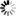Normal view

# Tensors, differential forms, and variational principles / by David Lovelock and Hanno Rund.

Material type:TextLanguage: English Publisher: New York : Dover, 1989Edition:  edDescription: xi, 366 sISBN: 9780486658407; 0486658406DDC classification: 515.63 Other classification: 53-01 | 49Q | Td | Tche | Telf
Contents:
Preliminary observations -- Affine tensor algebra in Euclidean geometry -- Tensor analysis on manifolds -- Additional topics from the tensor calculus -- The calculus of differential forms -- Invariant problems in the calculus of variations -- Riemannian geometry -- Invariant variational principles and physical field theories -- Appendix: Tensors and forms on differentiable manifolds
Summary: The aim of this book is to present a self-contained, reasonably modern account of tensor analysis and the calculus of exterior differential forms, adapted to the needs of physicists, engineers, and applied mathematicians. In the later, increasingly sophisticated chapters, the interaction between the concept of invariance and the calculus of variations is examined. This interaction is of profound importance to all physical field theories. Beginning with simple physical examples, the theory of tensors and forms is developed by a process of successive abstractions. This enables the reader to infer generalized principles from concrete situations - departing from the traditional approach to tensors and forms in terms of purely differential-geometric concepts. The treatment of the calculus of variations of single and multiple integrals is based ab initio on Carathéodory's method of equivalent integrals. Subsequent material explores the effects of invariance postulates on variational principles, focusing ultimately on relativistic field theories. Other discussions include: integral invariants; simple and direct derivations of Noether's theorems; and Riemannian spaces with indefinite metrics. The emphasis in this book is on analytical techniques, with abundant problems, ranging from routine manipulative exercises to technically difficult problems encountered by those using tensor techniques in research activities. A special effort has been made to collect many useful results of a technical nature, not generally discussed in the standard literature. The Appendix, newly revised and enlarged for the Dover edition, presents a reformulation of the principal concepts of the main text within the terminology of current global differential geometry, thus bridging the gap between classical tensor analysis and the fundamentals of more recent global theories.Other editions:
Star ratingsAverage rating: 0.0 (0 votes)
Holdings
Item type Current library Call number Status Date due Barcode
Book (loan)
515 Available 02400003367

Unabridged, corrected republ., first publ. 1975 by Wiley

Preliminary observations -- Affine tensor algebra in Euclidean geometry -- Tensor analysis on manifolds -- Additional topics from the tensor calculus -- The calculus of differential forms -- Invariant problems in the calculus of variations -- Riemannian geometry -- Invariant variational principles and physical field theories -- Appendix: Tensors and forms on differentiable manifolds

The aim of this book is to present a self-contained, reasonably modern account of tensor analysis and the calculus of exterior differential forms, adapted to the needs of physicists, engineers, and applied mathematicians. In the later, increasingly sophisticated chapters, the interaction between the concept of invariance and the calculus of variations is examined. This interaction is of profound importance to all physical field theories. Beginning with simple physical examples, the theory of tensors and forms is developed by a process of successive abstractions. This enables the reader to infer generalized principles from concrete situations - departing from the traditional approach to tensors and forms in terms of purely differential-geometric concepts. The treatment of the calculus of variations of single and multiple integrals is based ab initio on Carathéodory's method of equivalent integrals. Subsequent material explores the effects of invariance postulates on variational principles, focusing ultimately on relativistic field theories. Other discussions include: integral invariants; simple and direct derivations of Noether's theorems; and Riemannian spaces with indefinite metrics. The emphasis in this book is on analytical techniques, with abundant problems, ranging from routine manipulative exercises to technically difficult problems encountered by those using tensor techniques in research activities. A special effort has been made to collect many useful results of a technical nature, not generally discussed in the standard literature. The Appendix, newly revised and enlarged for the Dover edition, presents a reformulation of the principal concepts of the main text within the terminology of current global differential geometry, thus bridging the gap between classical tensor analysis and the fundamentals of more recent global theories.

Adress: Biblioteket, Blekinge Tekniska Högskola, 371 79 Karlskrona
Telefon: 0455 - 38 51 01
E-post: biblioteket@bth.se
Ansvarig för sidan: Biblioteket